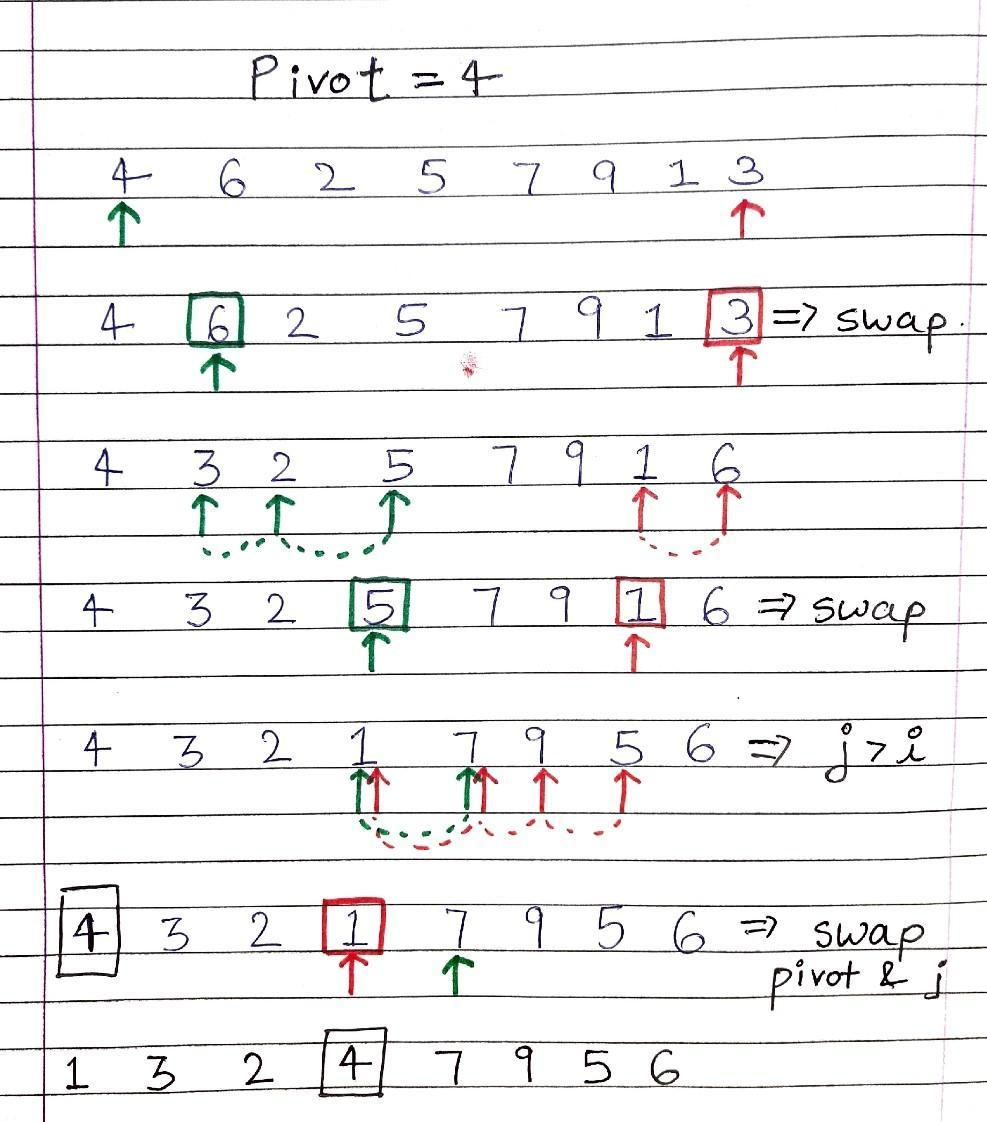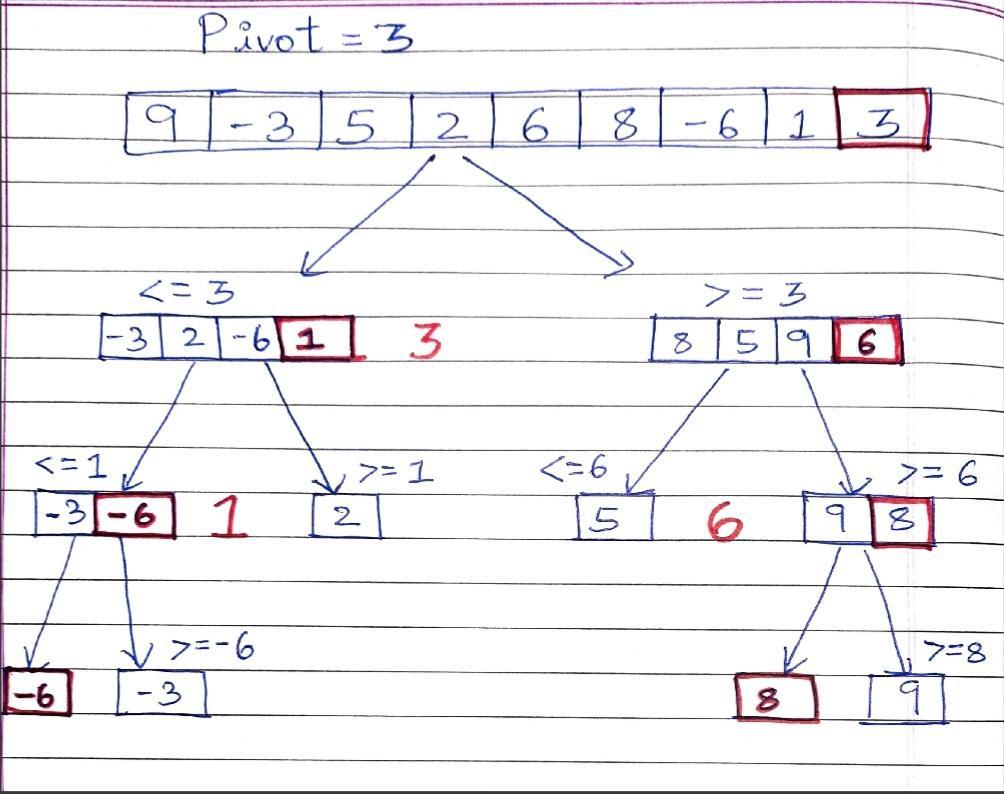# Quick Sort Algorithm

Problem Statement:  Given an array of n integers, sort the array using the Quicksort method.

Examples:

```Example 1:
Input:  N = 5  , Arr[] = {4,1,7,9,3}
Output: 1 3 4 7 9

Explanation: After sorting the array becomes 1, 3, 4, 7, 9

Example 2:
Input: N = 8 , Arr[] = {4,6,2,5,7,9,1,3}
Output: 1 2 3 4 5 6 7 9
Explanation: After sorting the array becomes 1, 3, 4, 7, 9```

Solution:

Disclaimer: Don’t jump directly to the solution, try it out yourself first.

Intuition:

-> First let’s understand what Quicksort is. It is a divide and conquer algorithm which can be used to sort an array of elements. An element is chosen ( namely as the pivot ) and the partition of the array is done to the left and the right of the pivot.

-> Pivot can be chosen in 4 different ways :

1. Median of Array
2. First element of the Array
3. Last element of the Array
4. Any Random element of the Array

-> The Main process of Quicksort is Partition. Once we decide on our pivot, we will put all values smaller than or equal to pivot in the left half and all the values greater than pivot in the right half of the pivot.

-> This means the pivot will be at its final sorted position after this process ends. Now we will recursively call to the left and right of the pivot so that the rest of the array also gets sorted.

-> The main intention of this process is to place the pivot at its final position, this is the position where the pivot will be in the final sorted array.The initial pivot is 3, at each call the red colored element is a new pivot elementApproach :

-> We will be using 2 pointers namely i, j. Initializing i at index 0 and j at index n-1 ( if the length of the array is n ).

-> We will swap arr[i] and arr[j] if  arr[i] > pivot  and arr[j] < pivot  and will increment i and decrement j . This goes on until i < j .

-> Finally when i > j, we will stop swapping arr[i] and arr[j] and swap pivot with arr[j] so that pivot gets its final position.

-> We will now recursively repeat this process in the left and right of the pivot so that we get our final sorted array.

Code:

## C++ Code

``````#include <bits/stdc++.h>
using namespace std;

class Solution
{
public:

void QuickSort(int arr[], int low, int high)
{
if (low < high) {

int pivot = partition(arr, low, high) ;
QuickSort(arr, low, pivot - 1)  ;
QuickSort(arr, pivot + 1, high)  ;
}
}

int partition (int arr[], int low, int high)
{
int pivot = arr[low]  ;
int i = low ;
int j = high ;

while (i < j) {

while (arr[i] <= pivot && i <= high - 1) {
i++  ;
}

while (arr[j] > pivot && j >= low) {
j-- ;
}

if (i < j)
swap(arr[i], arr[j])  ;
}

swap(arr[j], arr[low]) ;

return j ;
}
};

void printArray(int arr[], int n)
{
for (int i = 0; i < n; i++) {
cout << arr[i] << " " ;
}
cout<<endl;
}

int main()
{

int arr[] = {4, 6, 2, 5, 7, 8, 1, 3}  ;
int n = sizeof(arr) / sizeof(arr)  ;
Solution obj;
cout<<"Before Quick Sort: "<<endl;
printArray(arr,n);
obj.QuickSort(arr, 0, n - 1);
cout << "After Quick Sort: "<<endl;
printArray(arr, n);
return 0;
}
``````

Output:

Before Quick Sort:
4 6 2 5 7 8 1 3
After Quick Sort:
1 2 3 4 5 6 7 8

Time Complexity :

Following recurrence relation can be written for Quick sort :

F(n) = F(k) + F(n-1-k)

Here k is the number of elements smaller or equal to pivot and n-1-k denotes elements greater than the pivot.

There can be 2 cases :

1. Worst Case – This case occurs when pivot is the greatest or smallest element of the array . If partition is done and the last element is pivot , then the worst case would be either increasing order of the array or decreasing order of the array.

Recurrence:

F(n) = F(0)+F(n-1)  or  F(n) = F(n-1) + F(0)

Worst Case : O(n^2)

1. Best Case – This case occurs when pivot is the middle element of the array.

Recurrence :

F(n) = 2F(n/2)

Best Case : O(nLogn)

Space Complexity:  O(n)

## Java Code

``````import java.util.*;

class Solution{

static int partition(int arr[], int low , int high){
int pivot = arr[low]  ;
int i = low ;
int j = high ;

while (i < j) {

while (arr[i] <= pivot && i <= high - 1) {
i++  ;
}

while (arr[j] > pivot && j >= low) {
j-- ;
}

if (i < j){
int t = arr[i]  ;
arr[i] = arr[j]  ;
arr[j] = t ;
}
}

int t = arr[j]  ;
arr[j] = arr[low]  ;
arr[low] = t ;

return j ;
}

static void quicksort(int arr[] , int low, int high){

if (low < high) {

int pivot = partition(arr, low, high) ;
quicksort(arr, low, pivot - 1)  ;
quicksort(arr, pivot + 1, high)  ;

}

}
public static void main(String[] args) {

int n=8 ;
int arr[] = {4, 6, 2, 5, 7, 8, 1, 3}  ;
System.out.println("Before Quick Sort: ");
for(int i=0; i < n ;i++){
System.out.print(arr[i]+" ")  ;
}
System.out.println();
quicksort(arr,0,n-1)  ;

System.out.println("Sorted Array: ") ;
for(int i=0; i < n ;i++){
System.out.print(arr[i]+" ")  ;
}
}
}
``````

Output:

Before Quick Sort:
4 6 2 5 7 8 1 3
After Quick Sort:
1 2 3 4 5 6 7 8

Time Complexity :

Following recurrence relation can be written for Quick sort :

F(n) = F(k) + F(n-1-k)

Here k is the number of elements smaller or equal to pivot and n-1-k denotes elements greater than the pivot.

There can be 2 cases :

1. Worst Case – This case occurs when pivot is the greatest or smallest element of the array . If partition is done and the last element is pivot , then the worst case would be either increasing order of the array or decreasing order of the array.

Recurrence:

F(n) = F(0)+F(n-1)  or  F(n) = F(n-1) + F(0)

Worst Case : O(n^2)

1. Best Case – This case occurs when pivot is the middle element of the array.

Recurrence :

F(n) = 2F(n/2)

Best Case : O(nLogn)

Space Complexity:  O(n)

Special thanks to Shreyas Vishwakarma for contributing to this article on takeUforward. If you also wish to share your knowledge with the takeUforward fam, please check out this article